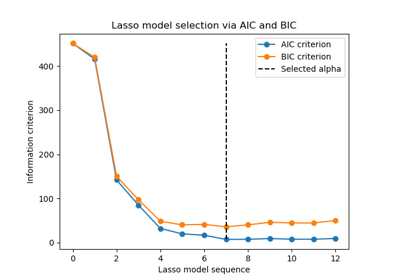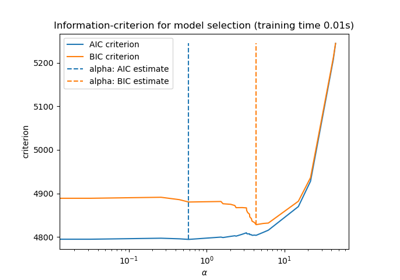# sklearn.linear_model.LassoLarsIC¶

class sklearn.linear_model.LassoLarsIC(criterion='aic', *, fit_intercept=True, verbose=False, normalize='deprecated', precompute='auto', max_iter=500, eps=2.220446049250313e-16, copy_X=True, positive=False, noise_variance=None)[source]

Lasso model fit with Lars using BIC or AIC for model selection.

The optimization objective for Lasso is:

(1 / (2 * n_samples)) * ||y - Xw||^2_2 + alpha * ||w||_1


AIC is the Akaike information criterion  and BIC is the Bayes Information criterion . Such criteria are useful to select the value of the regularization parameter by making a trade-off between the goodness of fit and the complexity of the model. A good model should explain well the data while being simple.

Read more in the User Guide.

Parameters:
criterion{‘aic’, ‘bic’}, default=’aic’

The type of criterion to use.

fit_interceptbool, default=True

Whether to calculate the intercept for this model. If set to false, no intercept will be used in calculations (i.e. data is expected to be centered).

verbosebool or int, default=False

Sets the verbosity amount.

normalizebool, default=False

This parameter is ignored when fit_intercept is set to False. If True, the regressors X will be normalized before regression by subtracting the mean and dividing by the l2-norm. If you wish to standardize, please use StandardScaler before calling fit on an estimator with normalize=False.

Changed in version 1.2: default changed from True to False in 1.2.

Deprecated since version 1.2: normalize was deprecated in version 1.2 and will be removed in 1.4.

precomputebool, ‘auto’ or array-like, default=’auto’

Whether to use a precomputed Gram matrix to speed up calculations. If set to 'auto' let us decide. The Gram matrix can also be passed as argument.

max_iterint, default=500

Maximum number of iterations to perform. Can be used for early stopping.

epsfloat, default=np.finfo(float).eps

The machine-precision regularization in the computation of the Cholesky diagonal factors. Increase this for very ill-conditioned systems. Unlike the tol parameter in some iterative optimization-based algorithms, this parameter does not control the tolerance of the optimization.

copy_Xbool, default=True

If True, X will be copied; else, it may be overwritten.

positivebool, default=False

Restrict coefficients to be >= 0. Be aware that you might want to remove fit_intercept which is set True by default. Under the positive restriction the model coefficients do not converge to the ordinary-least-squares solution for small values of alpha. Only coefficients up to the smallest alpha value (alphas_[alphas_ > 0.].min() when fit_path=True) reached by the stepwise Lars-Lasso algorithm are typically in congruence with the solution of the coordinate descent Lasso estimator. As a consequence using LassoLarsIC only makes sense for problems where a sparse solution is expected and/or reached.

noise_variancefloat, default=None

The estimated noise variance of the data. If None, an unbiased estimate is computed by an OLS model. However, it is only possible in the case where n_samples > n_features + fit_intercept.

New in version 1.1.

Attributes:
coef_array-like of shape (n_features,)

parameter vector (w in the formulation formula)

intercept_float

independent term in decision function.

alpha_float

the alpha parameter chosen by the information criterion

alphas_array-like of shape (n_alphas + 1,) or list of such arrays

Maximum of covariances (in absolute value) at each iteration. n_alphas is either max_iter, n_features or the number of nodes in the path with alpha >= alpha_min, whichever is smaller. If a list, it will be of length n_targets.

n_iter_int

number of iterations run by lars_path to find the grid of alphas.

criterion_array-like of shape (n_alphas,)

The value of the information criteria (‘aic’, ‘bic’) across all alphas. The alpha which has the smallest information criterion is chosen, as specified in .

noise_variance_float

The estimated noise variance from the data used to compute the criterion.

New in version 1.1.

n_features_in_int

Number of features seen during fit.

New in version 0.24.

feature_names_in_ndarray of shape (n_features_in_,)

Names of features seen during fit. Defined only when X has feature names that are all strings.

New in version 1.0.

lars_path

Compute Least Angle Regression or Lasso path using LARS algorithm.

lasso_path

Compute Lasso path with coordinate descent.

Lasso

Linear Model trained with L1 prior as regularizer (aka the Lasso).

LassoCV

Lasso linear model with iterative fitting along a regularization path.

LassoLars

Lasso model fit with Least Angle Regression a.k.a. Lars.

LassoLarsCV

Cross-validated Lasso, using the LARS algorithm.

sklearn.decomposition.sparse_encode

Sparse coding.

Notes

The number of degrees of freedom is computed as in .

To have more details regarding the mathematical formulation of the AIC and BIC criteria, please refer to User Guide.

References

Examples

>>> from sklearn import linear_model
>>> reg = linear_model.LassoLarsIC(criterion='bic')
>>> X = [[-2, 2], [-1, 1], [0, 0], [1, 1], [2, 2]]
>>> y = [-2.2222, -1.1111, 0, -1.1111, -2.2222]
>>> reg.fit(X, y)
LassoLarsIC(criterion='bic')
>>> print(reg.coef_)
[ 0.  -1.11...]


Methods

 fit(X, y[, copy_X]) Fit the model using X, y as training data. Get metadata routing of this object. get_params([deep]) Get parameters for this estimator. Predict using the linear model. score(X, y[, sample_weight]) Return the coefficient of determination of the prediction. set_fit_request(*[, copy_X]) Request metadata passed to the fit method. set_params(**params) Set the parameters of this estimator. set_score_request(*[, sample_weight]) Request metadata passed to the score method.
fit(X, y, copy_X=None)[source]

Fit the model using X, y as training data.

Parameters:
Xarray-like of shape (n_samples, n_features)

Training data.

yarray-like of shape (n_samples,)

Target values. Will be cast to X’s dtype if necessary.

copy_Xbool, default=None

If provided, this parameter will override the choice of copy_X made at instance creation. If True, X will be copied; else, it may be overwritten.

Returns:
selfobject

Returns an instance of self.

Get metadata routing of this object.

Please check User Guide on how the routing mechanism works.

Returns:

A MetadataRequest encapsulating routing information.

get_params(deep=True)[source]

Get parameters for this estimator.

Parameters:
deepbool, default=True

If True, will return the parameters for this estimator and contained subobjects that are estimators.

Returns:
paramsdict

Parameter names mapped to their values.

predict(X)[source]

Predict using the linear model.

Parameters:
Xarray-like or sparse matrix, shape (n_samples, n_features)

Samples.

Returns:
Carray, shape (n_samples,)

Returns predicted values.

score(X, y, sample_weight=None)[source]

Return the coefficient of determination of the prediction.

The coefficient of determination $$R^2$$ is defined as $$(1 - \frac{u}{v})$$, where $$u$$ is the residual sum of squares ((y_true - y_pred)** 2).sum() and $$v$$ is the total sum of squares ((y_true - y_true.mean()) ** 2).sum(). The best possible score is 1.0 and it can be negative (because the model can be arbitrarily worse). A constant model that always predicts the expected value of y, disregarding the input features, would get a $$R^2$$ score of 0.0.

Parameters:
Xarray-like of shape (n_samples, n_features)

Test samples. For some estimators this may be a precomputed kernel matrix or a list of generic objects instead with shape (n_samples, n_samples_fitted), where n_samples_fitted is the number of samples used in the fitting for the estimator.

yarray-like of shape (n_samples,) or (n_samples, n_outputs)

True values for X.

sample_weightarray-like of shape (n_samples,), default=None

Sample weights.

Returns:
scorefloat

$$R^2$$ of self.predict(X) w.r.t. y.

Notes

The $$R^2$$ score used when calling score on a regressor uses multioutput='uniform_average' from version 0.23 to keep consistent with default value of r2_score. This influences the score method of all the multioutput regressors (except for MultiOutputRegressor).

set_fit_request(*, copy_X: Union[bool, None, str] = '$UNCHANGED$') [source]

Request metadata passed to the fit method.

Note that this method is only relevant if enable_metadata_routing=True (see sklearn.set_config). Please see User Guide on how the routing mechanism works.

The options for each parameter are:

• True: metadata is requested, and passed to fit if provided. The request is ignored if metadata is not provided.

• False: metadata is not requested and the meta-estimator will not pass it to fit.

• None: metadata is not requested, and the meta-estimator will raise an error if the user provides it.

• str: metadata should be passed to the meta-estimator with this given alias instead of the original name.

The default (sklearn.utils.metadata_routing.UNCHANGED) retains the existing request. This allows you to change the request for some parameters and not others.

New in version 1.3.

Note

This method is only relevant if this estimator is used as a sub-estimator of a meta-estimator, e.g. used inside a Pipeline. Otherwise it has no effect.

Parameters:
copy_Xstr, True, False, or None, default=sklearn.utils.metadata_routing.UNCHANGED

Metadata routing for copy_X parameter in fit.

Returns:
selfobject

The updated object.

set_params(**params)[source]

Set the parameters of this estimator.

The method works on simple estimators as well as on nested objects (such as Pipeline). The latter have parameters of the form <component>__<parameter> so that it’s possible to update each component of a nested object.

Parameters:
**paramsdict

Estimator parameters.

Returns:
selfestimator instance

Estimator instance.

set_score_request(*, sample_weight: Union[bool, None, str] = '$UNCHANGED$') [source]

Request metadata passed to the score method.

Note that this method is only relevant if enable_metadata_routing=True (see sklearn.set_config). Please see User Guide on how the routing mechanism works.

The options for each parameter are:

• True: metadata is requested, and passed to score if provided. The request is ignored if metadata is not provided.

• False: metadata is not requested and the meta-estimator will not pass it to score.

• None: metadata is not requested, and the meta-estimator will raise an error if the user provides it.

• str: metadata should be passed to the meta-estimator with this given alias instead of the original name.

The default (sklearn.utils.metadata_routing.UNCHANGED) retains the existing request. This allows you to change the request for some parameters and not others.

New in version 1.3.

Note

This method is only relevant if this estimator is used as a sub-estimator of a meta-estimator, e.g. used inside a Pipeline. Otherwise it has no effect.

Parameters:
sample_weightstr, True, False, or None, default=sklearn.utils.metadata_routing.UNCHANGED

Metadata routing for sample_weight parameter in score.

Returns:
selfobject

The updated object.

## Examples using sklearn.linear_model.LassoLarsIC¶Lasso model selection via information criteria

Lasso model selection via information criteriaLasso model selection: AIC-BIC / cross-validation

Lasso model selection: AIC-BIC / cross-validation